EP3285100B1 - Optical fiber design method - Google Patents

Info

Publication number
EP3285100B1
EP3285100B1 EP16779871.9A EP16779871A EP3285100B1 EP 3285100 B1 EP3285100 B1 EP 3285100B1 EP 16779871 A EP16779871 A EP 16779871A EP 3285100 B1 EP3285100 B1 EP 3285100B1
Authority
EP
European Patent Office
Prior art keywords
λ
pcf
propagation
mode
eff
Prior art date
Legal status (The legal status is an assumption and is not a legal conclusion. Google has not performed a legal analysis and makes no representation as to the accuracy of the status listed.)
Active
Application number
EP16779871.9A
Other languages
German (de)
French (fr)
Other versions
EP3285100A1 (en
EP3285100A4 (en
Inventor
Takashi Matsui
Kyozo TSUJIKAWA
Fumihiko Yamamoto
Nobutomo HANZAWA
Current Assignee (The listed assignees may be inaccurate. Google has not performed a legal analysis and makes no representation or warranty as to the accuracy of the list.)
Nippon Telegraph and Telephone Corp
Original Assignee
Nippon Telegraph and Telephone Corp
Priority date (The priority date is an assumption and is not a legal conclusion. Google has not performed a legal analysis and makes no representation as to the accuracy of the date listed.)
Filing date
Publication date
Priority to JP2015082511 priority Critical
Priority to JP2015185252 priority
Application filed by Nippon Telegraph and Telephone Corp filed Critical Nippon Telegraph and Telephone Corp
Priority to PCT/JP2016/058864 priority patent/WO2016167080A1/en
Publication of EP3285100A1 publication Critical patent/EP3285100A1/en
Publication of EP3285100A4 publication Critical patent/EP3285100A4/en
Application granted granted Critical
Publication of EP3285100B1 publication Critical patent/EP3285100B1/en
Application status is Active legal-status Critical
Anticipated expiration legal-status Critical

• 238000001069 Raman spectroscopy Methods 0 claims description 13
• 239000003570 air Substances 0 claims description 79
• 238000004458 analytical methods Methods 0 description 5
• 238000005452 bending Methods 0 claims description 113
• 230000000903 blocking Effects 0 description 1
• 230000015556 catabolic process Effects 0 description 1
• 238000005253 cladding Methods 0 description 10
• 230000001276 controlling effects Effects 0 description 2
• 239000011162 core materials Substances 0 description 15
• 230000000875 corresponding Effects 0 description 2
• 230000001808 coupling Effects 0 description 1
• 238000010168 coupling process Methods 0 description 1
• 238000005859 coupling reaction Methods 0 description 1
• 238000006731 degradation Methods 0 description 1
• 230000004059 degradation Effects 0 description 1
• 230000000694 effects Effects 0 description 3
• 238000005516 engineering processes Methods 0 description 1
• 239000000835 fiber Substances 0 claims description 110
• 230000014509 gene expression Effects 0 claims description 6
• 239000003365 glass fiber Substances 0 claims description title 68
• 239000011799 hole materials Substances 0 claims description 80
• 230000001976 improved Effects 0 description 1
• 230000001965 increased Effects 0 claims description 10
• 230000003993 interaction Effects 0 claims description 3
• 239000010410 layers Substances 0 description 4
• 239000000463 materials Substances 0 description 3
• 239000002609 media Substances 0 description 3
• 238000000034 methods Methods 0 description 9
• 230000003287 optical Effects 0 description 13
• 229910052760 oxygen Inorganic materials 0 description 5
• 239000004038 photonic crystal Substances 0 claims description 13
• 239000000047 products Substances 0 description 1
• 230000000644 propagated Effects 0 claims description 37
• 230000001902 propagating Effects 0 claims description 15
• 239000010453 quartz Substances 0 description 2
• 229910052904 quartz Inorganic materials 0 description 2
• 230000002829 reduced Effects 0 description 3
• 230000001603 reducing Effects 0 description 1
• 238000006722 reduction reaction Methods 0 description 1
• 229910001885 silicon dioxide Inorganic materials 0 description 2
• 239000007787 solids Substances 0 description 4
• 230000000638 stimulation Effects 0 description 1
• 229910001890 sulfur dioxide Inorganic materials 0 description 3
• 229910001891 sulfur trioxide Inorganic materials 0 description 2
• 230000001629 suppression Effects 0 description 4
• 201000001845 syndromic X-linked intellectual disability Snyder type Diseases 0 claims 1

Images

•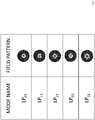•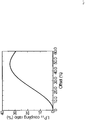•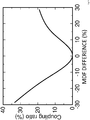•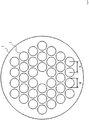•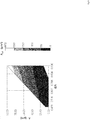•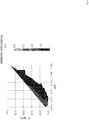•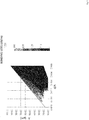•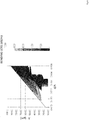•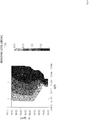•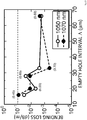•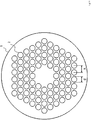•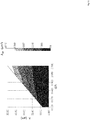•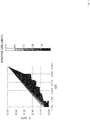•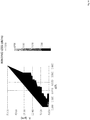•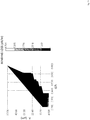•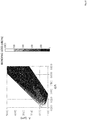••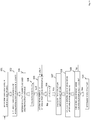•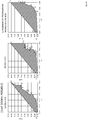•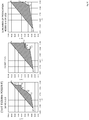•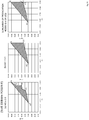•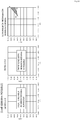•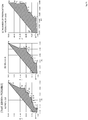•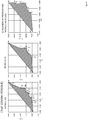•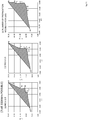••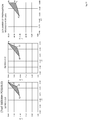••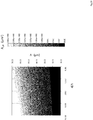•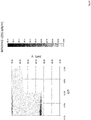•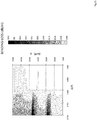•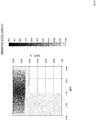•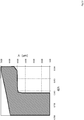Classifications

• GPHYSICS
• G02OPTICS
• G02BOPTICAL ELEMENTS, SYSTEMS, OR APPARATUS
• G02B6/00Light guides
• G02B6/02Optical fibre with cladding with or without a coating
• G02B6/028Optical fibre with cladding with or without a coating with core or cladding having graded refractive index
• G02B6/0288Multimode fibre, e.g. graded index core for compensating modal dispersion
• GPHYSICS
• G02OPTICS
• G02BOPTICAL ELEMENTS, SYSTEMS, OR APPARATUS
• G02B6/00Light guides
• G02B6/02Optical fibre with cladding with or without a coating
• G02B6/02004Optical fibre with cladding with or without a coating characterised by the core effective area or mode field radius
• G02B6/02009Large effective area or mode field radius, e.g. to reduce nonlinear effects in single mode fibres
• GPHYSICS
• G02OPTICS
• G02BOPTICAL ELEMENTS, SYSTEMS, OR APPARATUS
• G02B6/00Light guides
• G02B6/02Optical fibre with cladding with or without a coating
• G02B6/02295Microstructured optical fibre
• G02B6/02314Plurality of longitudinal structures extending along optical fibre axis, e.g. holes
• GPHYSICS
• G02OPTICS
• G02BOPTICAL ELEMENTS, SYSTEMS, OR APPARATUS
• G02B6/00Light guides
• G02B6/02Optical fibre with cladding with or without a coating
• G02B6/02295Microstructured optical fibre
• G02B6/02314Plurality of longitudinal structures extending along optical fibre axis, e.g. holes
• G02B6/02319Plurality of longitudinal structures extending along optical fibre axis, e.g. holes characterised by core or core-cladding interface features
• G02B6/02333Core having higher refractive index than cladding, e.g. solid core, effective index guiding
• GPHYSICS
• G02OPTICS
• G02BOPTICAL ELEMENTS, SYSTEMS, OR APPARATUS
• G02B6/00Light guides
• G02B6/02Optical fibre with cladding with or without a coating
• G02B6/02295Microstructured optical fibre
• G02B6/02314Plurality of longitudinal structures extending along optical fibre axis, e.g. holes
• G02B6/02342Plurality of longitudinal structures extending along optical fibre axis, e.g. holes characterised by cladding features, i.e. light confining region
• G02B6/02357Property of longitudinal structures or background material varies radially and/or azimuthally in the cladding, e.g. size, spacing, periodicity, shape, refractive index, graded index, quasiperiodic, quasicrystals
• GPHYSICS
• G02OPTICS
• G02BOPTICAL ELEMENTS, SYSTEMS, OR APPARATUS
• G02B6/00Light guides
• G02B6/02Optical fibre with cladding with or without a coating
• G02B6/02295Microstructured optical fibre
• G02B6/02314Plurality of longitudinal structures extending along optical fibre axis, e.g. holes
• G02B6/02342Plurality of longitudinal structures extending along optical fibre axis, e.g. holes characterised by cladding features, i.e. light confining region
• G02B6/02361Longitudinal structures forming multiple layers around the core, e.g. arranged in multiple rings with each ring having longitudinal elements at substantially the same radial distance from the core, having rotational symmetry about the fibre axis
• GPHYSICS
• G02OPTICS
• G02BOPTICAL ELEMENTS, SYSTEMS, OR APPARATUS
• G02B6/00Light guides
• G02B6/02Optical fibre with cladding with or without a coating
• G02B6/02295Microstructured optical fibre
• G02B6/02314Plurality of longitudinal structures extending along optical fibre axis, e.g. holes
• G02B6/02342Plurality of longitudinal structures extending along optical fibre axis, e.g. holes characterised by cladding features, i.e. light confining region
• G02B6/02366Single ring of structures, e.g. "air clad"
• GPHYSICS
• G02OPTICS
• G02BOPTICAL ELEMENTS, SYSTEMS, OR APPARATUS
• G02B6/00Light guides
• G02B6/02Optical fibre with cladding with or without a coating
• G02B6/02295Microstructured optical fibre
• G02B6/02314Plurality of longitudinal structures extending along optical fibre axis, e.g. holes
• G02B6/02342Plurality of longitudinal structures extending along optical fibre axis, e.g. holes characterised by cladding features, i.e. light confining region
• G02B6/02371Cross section of longitudinal structures is non-circular
• GPHYSICS
• G02OPTICS
• G02BOPTICAL ELEMENTS, SYSTEMS, OR APPARATUS
• G02B6/00Light guides
• G02B6/02Optical fibre with cladding with or without a coating
• G02B6/028Optical fibre with cladding with or without a coating with core or cladding having graded refractive index
• G02B6/0283Graded index region external to the central core segment, e.g. sloping layer or triangular or trapezoidal layer
• GPHYSICS
• G02OPTICS
• G02BOPTICAL ELEMENTS, SYSTEMS, OR APPARATUS
• G02B6/00Light guides
• G02B6/02Optical fibre with cladding with or without a coating
• G02B6/02295Microstructured optical fibre
• G02B6/02314Plurality of longitudinal structures extending along optical fibre axis, e.g. holes
• G02B6/02342Plurality of longitudinal structures extending along optical fibre axis, e.g. holes characterised by cladding features, i.e. light confining region
• G02B6/02347Longitudinal structures arranged to form a regular periodic lattice, e.g. triangular, square, honeycomb unit cell repeated throughout cladding

Description

Technical Field
• The present invention relates to a method of designing an optical fiber propagating light of high output and high quality.
• Background Art
• At present, there are two types of optical fibers, namely, single-mode and multi-mode optical fibers used in the field of laser processing by using a fiber laser. In a single-mode optical fiber used in laser processing, it is generally considered that a value of M2 as an index of beam quality is 2 or less. Therefore, since the beam quality of the single-mode optical fiber used to propagate emitted light of the fiber laser is better than that of the multi-mode optical fiber, the processing merit is great. However, an output power and a propagatable distance are limited by a nonlinear optical phenomenon, particularly, stimulated Raman scattering (SRS), and, for example, in the case of propagating a light wave with 1 kW or more, the propagatable distance is limited to several meters. For this reason, in some cases, the output power of the fiber laser may be propagated from several tens of meters to several hundreds of meters by using a multi-mode optical fiber and may be used for laser processing in some cases. However, in multi-mode fiber lasers, the beam quality and the value of M2 as an index of the beam quality are inevitably degraded in comparison with single-mode fiber lasers.
• In addition, as disclosed in Non Patent Literature 1, a single-mode fiber laser capable of obtaining an output power of about 10 kW is realized, however the length of the delivery fiber (feeding fiber) is limited to several meters. This is because, in order to suppress the SRS with the single-mode optical fiber, it is necessary to enlarge an effective area (Aeff) as described later, but at the same time, since the expansion is a tradeoff with the increase of a bending loss, it is necessary to expand an outer diameter of the delivery optical fiber to the order of millimeters to realize a much larger Aeff. If the outer diameter is increased in this manner, flexibility of the optical fiber is lost, it is difficult to bend the optical fiber, and thus, there is a problem that damage or breakage easily occurs and the optical fiber is not suitable for long-distance delivery.
• To summarize the above, in the related art, it is very difficult to propagate kW-class high power light with a single-mode optical fiber and a fiber laser from several tens of meters to several hundreds of meters.
• In order to solve this problem, it is effective to enlarge the effective area Aeff (which is generally proportional to the square of a mode field diameter) which is one of parameters of the optical fiber, and for example, design of a single-mode optical fiber with various refractive index distributions disclosed in Non Patent Literatures 2 to 5 has been studied.
• However, although the values of Aeff in the optical fiber disclosed in the cited literatures are prescribed, a propagation distance usable with respect to the output power and the like are not clarified, and it is not obvious whether the kW-class high power light can be propagated from several tens to several hundreds of meters. Furthermore, a range of the outer diameter of the optical fiber considering the flow of the fiber design and the practicality are not clarified.
• Citation List Non Patent Literature
• Patent Literature 1: US 2010/157418 A1 describes an optical fiber which may include cladding features that include a material (e.g., fluorine-doped silica glass) that may produce a very low relative refractive index difference with respect to cladding material in which the cladding features are disposed.
• Summary of Invention Technical Problem
• As described above, the structure of the optical fiber that can satisfy the requirements for an output power, a propagation distance, and a beam quality required in the examination examples in the related art has not been clarified.
• Therefore, an object of the present invention is to provide an optical fiber design method of designing an optical fiber that can satisfy desired requirements of an output power, a propagation distance, and a beam quality and the optical fiber.
• Solution to Problem
• The invention is defined by the features of the independent claim.
• In order to achieve the above-described object, Aeff is calculated from desired specifications of an optical fiber, a fiber structure is provisionally determined, and it is decided that the fiber structure is corrected in consideration of a relationship with a bending loss value in a fundamental mode or higher-order mode.
• More specifically, the optical fiber design method according to the present invention includes:
• a specification value determining step of determining a wavelength of propagating light, the fiber transmission loss of a photonic crystal fiber, PCF, at the wavelength of propagating light and a Raman gain coefficient of the PCF, a beam quality M2 after PCF propagation, a laser output power value, a propagation distance, and a minimum bending radius;
• maximum number of propagation modes calculating step of calculating the largest number n of propagation modes that can be propagated by using Mathematical Formula 1;
• an effective area calculating step of calculating an effective area Aeff from the fiber transmission loss and the Raman gain coefficient by using Mathematical Formula 2;
• a fiber structure calculating step of calculating diameter d and interval Λ of air holes of the PCF satisfying the Aeff;
• a bending loss calculating step of calculating a bending loss in the fundamental mode at the minimum bending radius in the PCF having a structure calculated in the fiber structure calculating step and calculating a bending loss in the fundamental mode at a propagation length from the propagation distance;
• a checking step of checking that the bending loss at the propagation length is less than a predetermined value and determining the structure of the PCF calculated in the fiber structure calculating step; and
• a mode increasing step of, in a case where the bending loss at the propagation length is equal to or more than the predetermined value in the checking step, repeating the fiber structure calculating step, the bending loss calculating step, and the checking step by increasing the number of modes by one until the number of modes reaches the number n of propagation modes.
• Mathematical Formulas 1 and 2 will be described later.
• In an optical fiber design method according to the present invention, a necessary Aeff of the optical fiber is calculated from requirements, a structure of a photonic crystal fiber (PCF) satisfying this is provisionally set, and the structure of the PCF is finely adjusted so that a bending loss becomes less than a specified value.
• In addition, the fiber structure may be determined by using the following method.
• Namely, in an optical fiber design method not according to the present invention includes:
• a specification value determining step of determining a fiber loss and Raman gain coefficient of a photonic crystal fiber (PCF) to be used, a wavelength of propagating light, a beam quality M2 after PCF propagation, a laser output power value, a propagation distance, and a minimum bending radius;
• maximum number of propagation modes calculating step of calculating the number n of propagation modes that can be propagated by using Mathematical Formula 1;
• an effective area calculating step of calculating an effective area Aeff from the fiber loss and the Raman gain coefficient by using Mathematical Formula 2;
• a fiber structure calculating step of calculating diameter d and interval Λ of air holes of the PCF having the Aeff or more and plotting points having the Aeff or more on a graph of which the horizontal axis is d/Λ and of which the vertical axis is Λ;
• a bending loss calculating step of calculating a bending loss at a minimum bending radius of a smallest higher-order mode cut off by the PCF from the diameter d and the interval Λ of the air holes of the PCF and plotting points having the bending loss of 1 dB/m or more on a graph of which the horizontal axis is d/Λ and of which the vertical axis is Λ; and
• a structure determining step of detecting an overlapping range where a region of the points plotted on the graph in the fiber structure calculating step and a region of the points plotted on the graph in the bending loss calculating step overlap each other and determining a PCF structure having air holes having diameters d and intervals Λ in the overlapping range.
• Mathematical Formulas 1 and 2 will be described later.
• In the design of the PCF, the PCF has air holes having diameters d and intervals Λ in an overlapping region where a region of Aeff of a desired value or more and a cutoff region in a desired higher order overlap each other on a graph where the horizontal axis represents d/Λ and the vertical axis represents Λ, so that it is possible to sufficiently cut off the mode which is the desired higher-order mode or more, and thus, it is possible to select a region where the Aeff is large.
• Therefore, according to the present invention, it is possible to provide an optical fiber design method capable of satisfying desired requirements of an output power, a propagation distance, and a beam quality.
• This is an example of a PCF designed by the optical fiber design method.
• The PCF is a PCF having a one-cell structure in which air holes of a diameter d are arranged at an interval Λ in a cross-section and has a feature in which, when the coordinates are represented as coordinates (d/Λ, Λ), air holes of a diameter d and an interval Λ that are in an area surrounded by a polygon having
• A1(0.42, 16.88),
• B(0.42, 10.94),
• C1(0.60, 15.63),
• C2(0.69, 31.88),
• D(0.74, 43.12),
• E(0.75, 44.38),
• C3(0.76, 47.81),
• F(0.81, 60.63),
• G(0.85, 60.63),
• H(0.85, 77.50), and
• I(0.90,91.88)
as its vertexes are included.
• Thus, Aeff can be configured to be as large as possible while the first high-order mode and higher modes are sufficiently cut off.
• The following is an example of a PCF that is designed using the above-described method of designing an optical fiber.
• The PCF is a PCF having a one-cell structure in which air holes of a diameter d are arranged at an interval Λ in a cross-section and has a feature in which, when the coordinates are represented as coordinates (d/Λ, Λ), air holes of a diameter d and an interval Λ that are in an area surrounded by a polygon having
• A1(0.42, 16.88),
• B(0.42, 10.94),
• C1(0.75, 15.00),
• D1(0.75, 20.00),
• C2(0.78, 35.00),
• D2(0.80, 35.93),
• E(0.80, 45.63),
• F(0.83, 51.56),
• C3(0.90, 54.38), and
• I(0.90,91.88)
as its vertexes are included.
• Thus, Aeff can be configured to be as large as possible while the third high-order mode and higher modes are sufficiently cut off.
• The following is another example of a PCF that is designed using the above-described method of designing an optical fiber.
• The PCF is a PCF having a one-cell structure in which air holes of a diameter d are arranged at an interval Λ in a cross-section and has a feature in which, when the coordinates are represented as coordinates (d/Λ, Λ), air holes of a diameter d and an interval Λ that are in an area surrounded by a polygon having
• A1(0.42, 16.88),
• B(0.42, 10.94),
• C(0.75, 14.24),
• D(0.75, 12.10),
• E(0.79, 20.00),
• F(0.85, 30.00),
• G(0.85, 41.58),
• H(0.89, 50.00),
• I(0.89, 58.95),
• J(0.90, 60.0), and
• K(0.90, 91.88)
as its vertexes are included.
• Thus, Aeff can be configured to be as large as possible while the fourth high-order mode and higher modes are sufficiently cut off.
• The following is another example of a PCF that is designed using the above-described method of designing an optical fiber.
• The PCF is a PCF having a seven-cell structure in which air holes of a diameter d are arranged at an interval Λ in a cross-section and has a feature in which, when the coordinates are represented as coordinates (d/Λ, Λ), air holes of a diameter d and an interval Λ that are in an area surrounded by a polygon having
• A1(0.20, 10.98),
• B(0.20, 4.95),
• C1(0.25, 5.27),
• D(0.29, 9.87),
• E(0.40, 12.25),
• F(0.40, 13.52),
• G(0.49, 14.15),
• H(0.49, 15.74),
• I(0.50, 18.12),
• J(0.58, 18.12),
• K(0.58, 19.86),
• C3(0.60, 20.34),
• L(0.60, 23.03),
• M(0.68, 23.99),
• N(0.68, 31.60),
• O(0.79, 48.73), and
• P(0.80, 50.00)
as its vertexes are included.
• Thus, Aeff can be configured to be as large as possible while the first high-order mode and higher modes are sufficiently cut off.
• The following is another example of a PCF that is designed using the above-described method of designing an optical fiber.
• The PCF is a PCF having a seven-cell structure in which air holes of a diameter d are arranged at an interval Λ in a cross-section and has a feature in which, when the coordinates are represented as coordinates (d/Λ, Λ), air holes of a diameter d and an interval Λ that are in an area surrounded by a polygon having
• A1(0.20, 10.98),
• B(0.20, 5.11),
• C1(0.40, 5.90),
• D(0.40, 10.03),
• E(0.50, 11.93),
• F(0.50, 14.47),
• C3(0.60, 20.18),
• G(0.69, 22.08),
• H(0.68, 23.67,),
• I(0.70, 24.30),
• J(0.70, 32.87), and
• K(0.80, 50.00)
as its vertexes are included.
• Thus, Aeff can be configured to be as large as possible while the third high-order mode and higher modes are sufficiently cut off.
• The following is another example of a PCF that is designed using the above-described method of designing an optical fiber.
• The PCF is a PCF having a seven-cell structure in which air holes of a diameter d are arranged at an interval Λ in a cross-section and has a feature in which, when the coordinates are represented as coordinates (d/Λ, Λ), air holes of a diameter d and an interval Λ that are in an area surrounded by a polygon having
• A1(0.20, 10.98),
• B(0.20, 5.11),
• C(0.50, 6.23),
• D(0.50, 10.00),
• E(0.60, 15.18),
• F(0.60, 17.76),
• G(0.65, 20.12),
• H(0.70, 20.35),
• I(0.79, 25.06),
• J(0.79, 29.53),
• K(0.78, 29.76),
• L(0.78, 38.29),
• M(0.80, 40.12), and
• N(0.80, 50.00)
as its vertexes are included.
• Thus, Aeff can be configured to be as large as possible while the fourth high-order mode and higher modes are sufficiently cut off.
• A light transmission using the above-described PCF will be described.
• It is the light transmission that propagates light with 1 kW or more from a laser for 10 m or more and outputting the light from the output end,
the laser and the output end are connected with a 2-mode fiber of which the number of propagation modes is 2 at the wavelength of the light, and
the light is propagated by setting an excitation ratio of the first higher-order mode of the 2-mode fiber to be 50% or less.
• The 2-mode fiber has larger Aeff compared with the single-mode fiber. For this reason, since the SRS can be suppressed, the propagation distance of the high-power light can be extended. Furthermore, since the excitation ratio of the first higher-order mode of the 2-mode fiber can be adjusted by the optical axis between the laser and the optical fiber, it is possible to transmit the high-power light with desired beam quality.
• More specifically, the light transmission according to the present invention is a light transmission of propagating light with 1 kW or more from a laser for 10 m or more and outputting the light from the output end,
the laser and the output end are connected with a 4-mode fiber of which the number of propagation modes is 4 or less at wavelength of the light, and
the light is propagated by setting an excitation ratio of the third higher-order mode of the 4-mode fiber to be 30% or less.
• The 4-mode fiber has larger Aeff compared with the single-mode fiber. For this reason, since the SRS can be suppressed, the propagation distance of high-power light can be extended. Furthermore, since the excitation ratio of the first higher-order mode and the second higher-order mode of the 4-mode fiber can be reduced by the optical axis between the laser and the optical fiber and the excitation ratio of the third higher-order mode can be adjusted by a mode field diameter of the optical fiber and a spot size of the light coupled to the optical fiber, it is possible to transmit the high power light with desired beam quality.
• Therefore, it is possible to realize a light transmission capable of satisfying desired requirements of an output power, a propagation distance, and a beam quality in a PCF designed by the optical fiber design method according to the present invention.
• Advantageous Effects of Invention
• According to the present invention, it is possible to provide an optical fiber design method of designing an optical fiber that can satisfy desired requirements of an output power, a propagation distance, and a beam quality and the optical fiber.
• Brief Description of Drawings
• Fig. 1 is a diagram that illustrates examples of waveguide modes of an optical fiber.
• Fig. 2 is a diagram that illustrates excitation efficiency with respect to an axial deviation from LP01 to LP11.
• Fig. 3 is a diagram that illustrates an example of an MFD difference of an optical fiber and efficiency of excitation from a fundamental mode to a third high-order mode.
• Fig. 4 is a diagram that illustrates an example of the cross-section of a PCF having a one-cell structure.
• Fig. 5 is a diagram that illustrates a relation among d/Λ, Λ, and Aeff satisfying a bending loss of 0.1 dB/m or less when light having a wavelength of 1070 nm is propagated in the fundamental mode in a PCF having the one-cell structure.
• Fig. 6 is a diagram that illustrates an area (cut-off area) in which a bending loss of a first high-order (LP11) mode of light having a wavelength of 1070 nm in a PCF having the one-cell structure is 1 dB/m or more.
• Fig. 7 is a diagram that illustrates an area (cut-off area) in which a bending loss of a second high-order (LP21) mode of light having a wavelength of 1070 nm in a PCF having the one-cell structure is 1 dB/m or more.
• Fig. 8 is a diagram that illustrates an area (cut-off area) in which a bending loss of a third high-order (LP02) mode of light having a wavelength of 1070 nm in a PCF having the one-cell structure is 1 dB/m or more.
• Fig. 9 is a diagram that illustrates an area (cut-off area) in which a bending loss of a fourth high-order (LP31) mode of light having a wavelength of 1070 nm in a PCF having the one-cell structure is 1 dB/m or more.
• Fig. 10 is a diagram that illustrates the structure dependency of the fundamental mode bending loss of a PCF having the one-cell structure for 1050 nm and 1070 nm.
• Fig. 11 is a diagram that illustrates an example of the cross-section of a PCF having a seven-cell structure.
• Fig. 12 is a diagram that illustrates a relation among d/Λ, Λ, and Aeff satisfying a bending loss of 0.1 dB/m or less when light having a wavelength of 1070 nm is propagated in the fundamental mode in a PCF having the seven-cell structure.
• Fig. 13 is a diagram that illustrates an area (cut-off area) in which a bending loss of the first high-order (LP11) mode of light having a wavelength of 1070 nm in a PCF having the seven-cell structure is 1 dB/m or more.
• Fig. 14 is a diagram that illustrates an area (cut-off area) in which a bending loss of the second high-order (LP21) mode of light having a wavelength of 1070 nm in a PCF having the seven-cell structure is 1 dB/m or more.
• Fig. 15 is a diagram that illustrates an area (cut-off area) in which a bending loss of the third high-order (LP02) mode of light having a wavelength of 1070 nm in a PCF having the seven-cell structure is 1 dB/m or more.
• Fig. 16 is a diagram that illustrates an area (cut-off area) in which a bending loss of the fourth high-order (LP31) mode of light having a wavelength of 1070 nm in a PCF having the seven-cell structure is 1 dB/m or more.
• Fig. 17 is a diagram that illustrates the structure dependency of the bending loss of the fundamental mode of a PCF having the seven-cell structure for 1050 nm and 1070 nm.
• Fig. 18 is a flowchart that illustrates a method of designing an optical fiber according to the present invention.
• Fig. 19 is a diagram that illustrates a relation among d/Λ, Λ, and Aeff (160 µm2 or more) satisfying (a) M2 ≤ 2.0, (b) M2 ≤ 3.3, and (c) the number of propagation modes is four or less when light having a wavelength of 1070 nm is propagated in a PCF having the one-cell structure. An area denoted by diagonal lines is an area in which 100 W output and 300 m propagation can be performed.
• Fig. 20 is a diagram that illustrates a relation among d/Λ, Λ, and Aeff (800 µm2 or more) satisfying (a) M2 ≤ 2.0, (b) M2 ≤ 3.3, and (c) the number of propagation modes is four or less when light having a wavelength of 1070 nm is propagated in a PCF having the one-cell structure. An area denoted by diagonal lines is an area in which 500 W output and 300 m propagation can be performed.
• Fig. 21 is a diagram that illustrates a relation among d/Λ, Λ, and Aeff (1600 µm2 or more) satisfying (a) M2 ≤ 2.0, (b) M2 ≤ 3.3, and (c) the number of propagation modes is four or less when light having a wavelength of 1070 nm is propagated in a PCF having the one-cell structure. An area denoted by diagonal lines is an area in which 1000 W output and 300 m propagation can be performed.
• Fig. 22 is a diagram that illustrates a relation among d/Λ, Λ, and Aeff (3200 µm2 or more) satisfying (a) M2 ≤ 2.0, (b) M2 ≤ 3.3, and (c) the number of propagation modes is four or less when light having a wavelength of 1070 nm is propagated in a PCF having the one-cell structure. An area denoted by diagonal lines is an area in which 600 kWm propagation can be performed. An area satisfying conditions (a) and (b) is not present.
• Fig. 23 is a diagram that illustrates a relation among d/Λ, Λ, and Aeff (160 µm2 or more) satisfying (a) M2 ≤ 2.0, (b) M2 ≤ 3.3, and (c) the number of propagation modes is four or less when light having a wavelength of 1070 nm is propagated in a PCF having the seven-cell structure. An area denoted by diagonal lines is an area in which 100 W output and 300 m propagation can be performed.
• Fig. 24 is a diagram that illustrates a relation among d/Λ, Λ, and Aeff (800 µm2 or more) satisfying (a) M2 ≤ 2.0, (b) M2 ≤ 3.3, and (c) the number of propagation modes is four or less when light having a wavelength of 1070 nm is propagated in a PCF having the seven-cell structure. An area denoted by diagonal lines is an area in which 500 W output and 300 m propagation can be performed.
• Fig. 25 is a diagram that illustrates a relation among d/Λ, Λ, and Aeff (1600 µm2 or more) satisfying (a) M2 ≤ 2.0, (b) M2 ≤ 3.3, and (c) the number of propagation modes is four or less when light having a wavelength of 1070 nm is propagated in a PCF having the seven-cell structure. An area denoted by diagonal lines is an area in which 1000 W output and 300 m propagation can be performed.
• Fig. 26 is a diagram that illustrates a relation among d/Λ, Λ, and Aeff (3200 µm2 or more) satisfying (a) M2 ≤ 2.0, (b) M2 ≤ 3.3, and (c) the number of propagation modes is four or less when light having a wavelength of 1070 nm is propagated in a PCF having the seven-cell structure. An area denoted by diagonal lines is an area in which 2000 W output and 300 m propagation can be performed.
• Fig. 27 is a diagram that illustrates a relation among d/Λ, Λ, and Aeff (4800 µm2 or more) satisfying (a) M2 ≤ 2.0, (b) M2 ≤ 3.3, and (c) the number of propagation modes is four or less when light having a wavelength of 1070 nm is propagated in a PCF having the seven-cell structure. An area denoted by diagonal lines is an area in which 3000 W output and 300 m propagation can be performed.
• Fig. 28 is a diagram that illustrates another example of the cross-section of a PCF having the one-cell structure.
• Fig. 29 is a diagram that illustrates a relation among d/Λ, Λ, and Aeff satisfying a bending loss of 0.1 dB/m or less when light having a wavelength of 1070 nm is propagated in a PCF having the one-cell structure.
• Fig. 30 is a diagram that illustrates an area (cut-off area) in which a bending loss of the first high-order (LP11) mode of light having a wavelength of 1070 nm in a PCF having the one-cell structure is 1 dB/m or more.
• Fig. 31 is a diagram that illustrates an area (cut-off area) in which a bending loss of the second high-order (LP21) mode of light having a wavelength of 1070 nm in a PCF having the one-cell structure is 1 dB/m or more.
• Fig. 32 is a diagram that illustrates an area (cut-off area) in which a bending loss of the third high-order (LP02) mode of light having a wavelength of 1070 nm in a PCF having the one-cell structure is 1 dB/m or more.
• Fig. 33 is a diagram that illustrates a relation between d/Λ and Λ satisfying M2 ≤ 2.0 when light having a wavelength of 1070 nm is propagated in a PCF having the one-cell structure.
Description of Embodiments
• Embodiments of the present invention will be described with reference to the attached drawings. The embodiments described below are examples of the present invention, and thus, the present invention is not limited to the embodiments presented below. In the following description and the drawings, like reference numerals denote like elements.
• (Embodiment 1)
• Characteristics of the present invention are to increase an output power and to extend a propagation distance by enlarging Aeff. A major difference of the present invention from the related art is that an optical fiber has a structure capable of propagating a plurality of modes. The present invention sufficiently reduces an efficiency at which the higher-order mode is excited in an input unit and clarifies a region where M2 can be made sufficiently small, so that Aeff can be set to a value that cannot be realized in the related art.
• First, the value of M2 that is an index of beam quality is described below. An embodiment of the present invention will be described based on each waveguide mode illustrated in Fig. 1.
• The M2 values for the waveguide modes of the optical fiber are disclosed to be 1.1 for the fundamental mode, 3.3 for the first higher-order mode, 3.3 for the second higher-order mode, and 3.1 for the third higher-order mode in Non Patent Literature 6. In addition, it is disclosed that, in a case where the fundamental mode and the first higher-order mode coexist, the M2 value varies depending on a phase relationship between an excitation ratio of the first higher-order mode and the fundamental mode, and it can be understood that, if the excitation ratio of the first higher-order mode is lower than about 50%, the M2 value becomes 2.0 or less.
• A first beam propagation method according to the embodiment is a light transmission of propagating light with 1 kW or more from a laser for 10 m or more and outputting the light from an output end,
the laser and the output end are connected with a 2-mode fiber of which the number of propagation modes is 2 at the wavelength of the light, and
the light is propagated by setting an excitation ratio of the first higher-order mode of the 2-mode fiber to be 50% or less.
• In the first higher-order mode, the excitation ratio varies depending on an amount of axis shift from the center of the optical fiber when light is generally input to the optical fiber. In addition, reduction of the excitation ratio of the first higher-order mode down to 50% or less can be sufficiently realized with existing optical input/output alignment (optical axis alignment) technology.
• Accordingly, if a 2-mode fiber in which the second higher-order mode is cut off and only the fundamental mode and the first higher-order mode exist is used, by appropriately controlling the excitation ratio, high-quality beam propagation with an M2 value of 2.0 or less which cannot be realized with the multi-mode fiber in the related art becomes possible, and fiber design greatly exceeding Aeff which cannot be realized with the single-mode fiber in the related art becomes possible. In addition, the worst value of the M2 value of the 2-mode fiber in which only the fundamental mode and the first higher-order mode exist is 3.3, and fiber design of enabling relatively high-quality beam propagation in comparison with the multi-mode optical fiber is possible.
• The basis of the worst value of the M2 value of the 2-mode fiber is illustrated in FIG. 4 of Non Patent Literature 6. In this figure, M2 denotes the excitation ratio of the LP11 mode of a case where the LP01 (fundamental mode) and the LP11 mode (first higher-order mode) coexist, and M2 is 3.3 when α (excitation ratio of the LP11 mode) is 1.0. In addition, unless the LP11 mode is intentionally excited, the LP11 mode will not be excited 100%, so that the worst value of the M2 value of the 2-mode fiber is 3.3.
• A second beam propagation method according to the embodiment is a light transmission of propagating light with 1 kW or more from a laser for 10 m or more and outputting the light from the output end,
the laser and the output end are connected with a 4-mode fiber of which the number of propagation modes is 4 at the wavelength of light, and
the light is propagated by setting an excitation ratio of the third higher-order mode of the 4-mode fiber to be 30% or less.
• Non Patent Literature 6 also discloses an M2 value when the fundamental mode and the third higher-order mode coexist, and thus, it can be understood that, if the excitation ratio of the third higher-order mode is lower than about 30%, the M2 value becomes 2 or less. The fundamental mode and the third higher-order mode are modes having the electric field peak at the center of the fiber, and the coupling efficiency varies depending on a spot size (MFD 2) of the light input to the fiber having a mode field diameter (MFD 1). FIG. 3 is a diagram illustrating a relationship between an MFD difference and an efficiency (rate of occurrence of the third higher-order mode) of excitation of the third higher-order mode when the vertical axis represents the efficiency of excitation of the third higher-order mode and the horizontal axis represents the MFD difference ((MFD2 - MFD1)/MFD1) input to the fiber.
• From FIG. 3, it is possible to reduce the occurrence of the third higher-order mode to about 30% or less by suppressing the MFD difference within 20% or by inputting a beam having a spot size more than the MFD 1 of the fiber, so that the M2 value can be reduced to 2 or less. More specifically, since the third higher-order mode occurs according to the distance between the optical fibers (corresponding to a difference in beam diameter between input light and a fundamental mode of a 4-mode optical fiber), the distance between the fiber laser and the 4-mode optical fiber is adjusted. In addition, the first higher-order mode and the second higher-order mode are higher-order modes which occur due to axis shift of the fiber laser and the optical fiber and can be sufficiently suppressed from results of an axis shift and an excitation amount of the first higher-order mode.
• From the above description, by appropriately controlling the excitation ratio by using the 4-mode fiber where the fourth higher-order mode is cut off, it is possible to design a fiber where high-quality beam propagation with an M2 value of 2.0 or less is possible.
Furthermore, since the worst value of the M2 value of the 4-mode fiber is 3.3, it is possible to design a fiber that enables relatively high-quality beam propagation with an M2 value of at worst 3.3 or less.
• In addition, the ground for the worst value of the M2 value of the 2-mode fiber is illustrated in FIG. 6 of Non Patent Literature 6. In this figure, M2 is illustrated with respect to the excitation ratio of LP02 mode in a case where LP01 (fundamental mode) and LP02 mode (third higher-order mode) coexist, and when α (excitation ratio of LP02 mode) is 0.9, the M2 is 3.3. Therefore, the worst value of the M2 value of the 4-mode fiber is 3.3.
• In the propagation of only the single-mode, there exists a limit to enlargement of Aeff, but as in the light transmission described in the embodiment, the propagation of several modes is permitted and the design range is widened by using a 2-mode fiber or a 4-mode fiber, so that it is possible to realize the Aeff which does not exist in the related art. Therefore, by using the light transmission according to the embodiment, it is possible to propagate light satisfying the desired requirements of an output power, a propagation distance, and a beam quality.
• (Embodiment 2)
• As an example not according to the invention this embodiment relates to a design method of structural parameters (diameters d of the air hole 2 and intervals A between the air holes 2) for realizing enlargement of an effective area Aeff and implementing a predetermined bending loss αb in a PCF having a 1-cell core structure having air holes 2 illustrated in FIG. 4. In the embodiment, a minimum bending radius in a fundamental mode and a bending radius defining an effective cutoff of a higher-order mode are described as 140 mm, but the method according to the embodiment is not limited thereto.
• The design method includes:
• a specification value determining step of determining fiber loss and Raman gain coefficient of a photonic crystal fiber (PCF) to be used, a wavelength of propagating light, a beam quality M2 after PCF propagation, a laser output power value, a propagation distance and a minimum bending radius;
• propagation modesmaximum number of propagation modes calculating step of calculating the number n of propagation modes that can be propagated by using Mathematical Formula 1;
• an effective area calculating step of calculating an effective area Aeff from the fiber loss and the Raman gain coefficient by using Mathematical Formula 2;
• a fiber structure calculating step of calculating diameter d and interval Λ of air holes of the PCF having the Aeff or more and plotting points having the Aeff or more on a graph of which the horizontal axis is d/Λ and of which the vertical axis is Λ;
• a bending loss calculating step of calculating a bending loss at a minimum bending radius of a smallest higher-order mode cut off by the PCF from the diameter d and the interval A of the air holes of the PCF and plotting points having the bending loss of 1 dB/m or more on a graph of which the horizontal axis is d/Λ and of which the vertical axis is Λ; and
• a structure determining step of detecting an overlapping range where a region of the points plotted on the graph in the fiber structure calculating step and a region of the points plotted on the graph in the bending loss calculating step overlap each other and determining a PCF structure having air holes having diameters d and intervals Λ in the overlapping range.
• Mathematical Formulas 1 and 2 will be described later.
• As illustrated in FIG. 4, the optical fiber according to the embodiment is a photonic crystal fiber (PCF) having a 1-cell structure which has a core portion and a cladding portion that surrounds the core portion, wherein the core portion and the cladding portion are made of a medium having a uniform optical refractive index, and a plurality of uniform air holes 2 are formed in the cladding portion in the longitudinal direction. In addition, the 1-cell structure in the present invention denotes a structure of a photonic crystal having one defect, in which only the air holes in the central portion of the photonic crystal formed with the air holes arranged in a triangular lattice shape are made of quartz.
• FIG. 5 is a diagram illustrating a range of the Aeff of the PCF in the region where the bending loss in the fundamental mode with a bending radius of 140 mm is 1.0 dB/m or less with respect to light having a wavelength of 1070 nm when the horizontal axis represents d/Λ and the vertical axis represents Λ. The bending radius and the bending loss value in the fundamental mode are not limited to the definition and the specified value according to the present invention, but it is possible to determine the parameters used for the design according to the required characteristics. The Aeff is obtained by using the following Mathematical Formula.
[Numerical Expression 3] $A eff = ∫ ∫ | E x y | 2 dxdy 2 ∫ ∫ | E x y | 4 dxdy$ Herein, E is the electric field of light, and x and y are the coordinates in the fiber cross section (assumed to be the xy plane).
• FIG. 6 illustrates the bending loss in the first higher-order mode at a bending radius of 140 mm of the 1-cell structure PCF with respect to light having a wavelength of 1070 nm when the horizontal axis represents d/Λ and the vertical axis represents Λ. The plotted range in the figure illustrates the bending loss in the first higher-order mode in the range of 1 dB/m or more (the range in which the cutoff is obtained). By setting to d/Λ and Λ where the range illustrated in FIG. 5 and the range illustrated in FIG. 6 overlap each other, the first higher-order mode or more is sufficiently cut off, and thus, it is possible to select a region where the Aeff is large. For example, when the coordinates are represented by (d/Λ, Λ), the air holes are set to d and Λ in a region surrounded by a polygon having four vertices
• A(0.42, 16),
• B(0.42, 10),
• C(0.53, 10), and
• D(0.80, 56).
• More specifically, it can be understood from FIG. 6 that, if d/Λ is 0.724 and Λ is about 45 µm, Aeff is 1400 µm2, and the bending loss in the first higher-order mode is 40 dB/m or more, namely, the structure which d/Λ is 0.724 and Λ is about 45 µm becomes a single mode effectively.
• FIG. 7 illustrates the bending loss (1 dB/m or more) in the second higher-order mode at a bending radius of 140 mm of the 1-cell structure PCF with respect to light having a wavelength of 1070 nm when the horizontal axis represents d/Λ and the vertical axis represents Λ. By setting to d/Λ and Λ where the range illustrated in FIG. 5 and the range illustrated in FIG. 7 overlap each other, the second higher-order mode or more is sufficiently cut off, and thus, it is possible to select a region where the Aeff is large. For example, when the coordinates are represented by (d/Λ, Λ), the air holes are set to d and Λ in a region surrounded by a polygon having four vertices
• A(0.42, 16),
• B(0.42, 10),
• C(0.76, 10), and
• D(0.80, 56).
• In addition, FIG. 8 illustrates the bending loss (1 dB/m or more) in the third higher-order mode at a bending radius of 140 mm of the 1-cell structure PCF with respect to light having a wavelength of 1070 nm when the horizontal axis represents d/Λ and the vertical axis represents Λ. By setting to d/Λ and Λ where the range illustrated in FIG. 5 and the range illustrated in FIG. 8 overlap each other, the third higher-order mode or more is sufficiently cut off, and thus, it is possible to select a region where the Aeff is large. For example, when the coordinates are represented by (d/Λ, Λ), the air holes are set to d and Λ in a region surrounded by a polygon having four vertices
• A(0.42, 16),
• B(0.42, 10),
• C(0.76, 10), and
• D(0.80, 56).
• In addition, FIG. 9 illustrates the bending loss (1 dB/m or more) in the fourth higher-order mode at a bending radius of 140 mm of the 1-cell structure PCF with respect to light having a wavelength of 1070 nm when the horizontal axis represents d/Λ and the vertical axis represents Λ. By setting to d/Λ and Λ where the range illustrated in FIG. 5 and the range illustrated in FIG. 9 overlap each other, the fourth higher-order mode or more is sufficiently cut off, and thus, it is possible to select a region where the Aeff is large. For example, when the coordinates are represented by (d/Λ, Λ), the air holes are set to d and Λ in a region surrounded by a polygon having four vertices
• A(0.42, 16),
• B(0.42, 10),
• C(0.76, 10), and
• D(0.80, 56).
• By setting to d/Λ and Λ where the range illustrated in FIG. 5 and the range illustrated in FIG. 7, FIG. 8 or FIG. 9 overlap each other as described above, the second higher-order mode or more, the third higher-order mode or more, or the fourth higher-order mode or more is sufficiently cut off, and thus, it is possible to select a region where the Aeff is large.
• FIG. 10 illustrates wavelength dependency of a bending loss of a PCF with a 1-cell structure. In the figure, the solid line (open circles) and the broken line (black circles) illustrate air hole interval (Λ) dependency of the bending loss in the fundamental mode for the wavelength 1050 nm and the wavelength 1070 nm, respectively, as, and the values in parentheses in the figure indicate values of d/Λ for each Λ. It is generally known that the PCF has characteristics in that the Aeff has almost the same value regardless of the propagation wavelength and the bending loss increases as the wavelength becomes shorter. FIG. 10 illustrates that the structure illustrated in this design is realized in any structure where the bending loss in the fundamental mode is 0.1 dB/m or less even at a wavelength of 1050 nm and that the present design is effective at a wavelength of 1050 nm or more.
• (Embodiment 3)
• As an example not according to the invention this embodiment relates to a design method of structural parameters (diameters d of the air hole 2 and intervals A between the air holes 2) for realizing enlargement of Aeff and implementing a predetermined bending loss αb in a PCF having a 7-cell core structure having a plurality of air holes 2 as illustrated in FIG. 11. This design method also employs the design method described in the second embodiment.
• As illustrated in FIG. 11, the optical fiber according to the embodiment is a PCF having a 7-cell structure which has a core portion and a cladding portion that surrounds the core portion, wherein the core portion and the cladding portion are made of a medium having a uniform optical refractive index, and a plurality of uniform air holes 2 are formed in the cladding portion in the longitudinal direction. In addition, the 7-cell structure in the present invention denotes a structure of a photonic crystal having seven defects, in which one air hole in the central portion of the photonic crystal formed with the air holes arranged in a triangular lattice shape and six air holes around the air hole are made of quartz.
• FIG. 12 is a diagram illustrating Aeff in a region where the bending loss in the fundamental mode at a bending radius of 140 mm is 1.0 dB/m or less when the horizontal axis represents d/Λ and the vertical axis represents Λ. Since the PCF according to the embodiment has a 7-cell structure, in comparison with the 3-layer structure of the 1-cell structure of Embodiment 1, it is advantageous in that the fiber outer diameter can be reduced in a region where Aeff is 1000 µm2 or more. In the 3-layer 1-cell structure, a cladding diameter of 500 µm is required to achieve the Aeff of 1000 µm2, whereas in the 4-layer 7-cell structure, it can be realized with an outer diameter of about 200 µm. The definition of the bending loss value and cutoff in the fundamental mode is not limited to the definition and the specified value of the present invention, but it is possible to determine parameters used for the design according to the required characteristics.
• FIG. 13 illustrates the bending loss in the first higher-order (LP11) mode at a bending radius of 140 mm of the 7-cell structure PCF with respect to light having a wavelength of 1070 nm when the horizontal axis represents d/Λ and the vertical axis represents Λ. The plotted range in the figure illustrates the bending loss in the first higher-order mode in the range of 1 dB/m or more (the range in which the cutoff is obtained). By setting to d/Λ and Λ where the range illustrated in FIG. 12 and the range illustrated in FIG. 13 overlap each other, the first higher-order mode or more is sufficiently cut off, and thus, it is possible to select a region where the Aeff is large. For example, when the coordinates are represented by (d/Λ, Λ), the air holes are set to d/Λ and Λ in a region surrounded by a triangle having three vertices
• A(0.20, 7.80),
• B(0.34, 10.82), and
• C(0.78, 48.42).
• More specifically, it can be understood that, if d/Λ is 0.68 and Λ is about 40 µm, Aeff is 5700 µm2 and the bending loss in the first higher-order mode is 20 dB/m or more, namely, the structure which d/Λ is 0.68 and Λ is about 40 µm becomes a single mode effectively.
• FIG. 14 illustrates the bending loss (1 dB/m or more) in the second higher-order mode at a bending radius of 140 mm of the 7-cell structure PCF with respect to light having a wavelength of 1070 nm when the horizontal axis represents d/Λ and the vertical axis represents Λ. By setting to d/Λ and Λ where the range illustrated in FIG. 12 and the range illustrated in FIG. 14 overlap each other, the second higher-order mode or more is sufficiently cut off, and thus, it is possible to select a region where the Aeff is large. For example, when the coordinates are represented by (d/Λ, Λ), the air holes are set to d/Λ and Λ in a region surrounded by a polygon having four vertices
• A(0.20, 7.80),
• B(0.20, 4.00),
• C(0.80, 4.00), and
• D(0.80, 50.0).
• In addition, FIG. 15 illustrates the bending loss (1 dB/m or more) in the third higher-order mode at a bending radius of 140 mm of the 1-cell structure PCF with respect to light having a wavelength of 1070 nm when the horizontal axis represents d/Λ and the vertical axis represents Λ. By setting to d/Λ and Λ where the range illustrated in FIG. 12 and the range illustrated in FIG. 15 overlap each other, the third higher-order mode or more is sufficiently cut off, and thus, it is possible to select a region where the Aeff is large. For example, when the coordinates are represented by (d/Λ, Λ), the air holes are set to d/Λ and Λ in a region surrounded by a polygon having four vertices
• A(0.20, 7. 80),
• B(0.20, 4.00),
• C(0.80, 4.00), and
• D(0.80, 50.0).
• In addition, FIG. 16 illustrates the bending loss (1 dB/m or more) in the fourth higher-order mode at a bending radius of 140 mm of the 1-cell structure PCF with respect to light having a wavelength of 1070 nm when the horizontal axis represents d/Λ and the vertical axis represents Λ. By setting to d/Λ and Λ where the range illustrated in FIG. 12 and the range illustrated in FIG. 16 overlap each other, the fourth higher-order mode or more is sufficiently cut off, and thus, it is possible to select a region where Aeff is large. For example, when the coordinates are represented by (d/Λ, Λ), the air holes are set to d/Λ and Λ in a region surrounded by a polygon having four vertices
• A(0.20, 7.80),
• B(0.20, 4.00),
• C(0.80, 4.00), and
• D(0.80, 50.0).
• As described above, by setting to d/Λ and Λ where the range illustrated in FIG. 12 and the range illustrated in FIG. 14, FIG. 15 or FIG. 16 overlap each other, the second higher-order mode or more, the third higher-order mode or more, or the fourth higher-order mode or more is sufficiently cut off, and thus, it is possible to select a region where the Aeff is large.
• FIG. 17 illustrates wavelength dependency of a bending loss of a PCF with a 7-cell structure. In the figure, the solid line (open circles) and the broken line (black circles) illustrate air hole interval Λ dependency of the bending loss in the fundamental mode for the wavelength 1050 nm and the wavelength 1070 nm, respectively, as, and the values in parentheses in the figure indicate values of d/Λ for each Λ. It is generally known that the PCF has characteristics in that the Aeff has almost the same value regardless of the propagation wavelength and the bending loss increases as the wavelength becomes shorter. FIG. 17 illustrates that the structure illustrated in this design is realized in any structure where the bending loss in the fundamental mode is 0.1 dB/m or less even at a wavelength of 1050 nm and that the present design is effective at a wavelength of 1050 nm or more.
• (Embodiment 4)
• In the embodiment, another design method of designing the 2-mode fiber or the 4-mode fiber described in Embodiments 1 to 3 on the basis of specifications as an optical propagation medium such as a laser processing system will be described. The optical fiber design method according to the embodiment includes:
• a specification value determining step of determining a wavelength of propagating light, the fiber transmission loss of a photonic crystal fiber, PCF, at the wavelength of propagating light, a beam quality M2 after PCF propagation, a laser output power value, a propagation distance, and a minimum bending radius;
• maximum number of propagation modes calculating step of calculating the largest number n of propagation modes that can be propagated by using Mathematical Formula 1;
• an effective area calculating step of calculating an effective area Aeff from the fiber loss and the Raman gain coefficient by using Mathematical Formula 2;
• a fiber structure calculating step of calculating diameter and interval of air holes of the PCF satisfying the Aeff;
• a bending loss calculating step of calculating a bending loss in the fundamental mode at the minimum bending radius in a PCF having a structure calculated in the fiber structure calculating step and calculating a bending loss in the fundamental mode at a propagation length from the propagation distance;
• a checking step of checking that the bending loss at the propagation length is, for example, less than 0.1 dB and determining the structure of the PCF calculated in the fiber structure calculating step; and
• a mode increasing step of, in a case where the bending loss at the propagation length is, for example, 0.1 dB or more in the checking step, repeating the fiber structure calculating step, the bending loss calculating step, and the checking step by increasing the number of modes by one until the number of modes reaches number n of propagation modes.
• FIG. 18 is a flowchart illustrating a procedure of the optical fiber design method according to the embodiment.
• First, in step S01 (specification value determining step), the used wavelength and target beam quality (M2 value) are determined. Next, in step S02 (number-of-propagation modes calculating step), the number of propagation modes that can satisfy the M2 value of step S01 is calculated from Mathematical Formula (1) (Mathematical Formula (17) of Non Patent Literature 6), and the number n of propagation modes is determined.
• After that, an output power and a propagation distance to be used in step S03 (specification value determining step) are determined, and in step S04 (effective area calculating step), a required effective area (Aeff) is calculated by using Mathematical Formula (2) (SRS threshold definition formula (8.1.13) disclosed in Non Patent Literature 7).
• Next, in step S05, the number (1 + k) of propagatable modes is determined. At this time, in order to improve the beam quality as much as possible, the design is started from the single mode of which the number (1 + k) of propagation modes is 1 (k = 0). Since step S06 can always be satisfied when k = 0, the process proceeds to step S07 (fiber structure calculating step), and a fiber structure satisfying the Aeff calculated in step S04 is calculated. In step S08 (bending loss calculating step), the bending loss in the fundamental mode at the minimum bending radius to be used in the fiber structure designed in step S07 is calculated, and in a case where the bending loss exceeds a specified value in step S09, the process returns to step S05, and the number of propagatable modes is incremented by 1, and the procedure up to step S09 is repeated (mode increasing step). At this time, in a case where the number (1 + k) of modes exceeds the number n of propagation modes in step S06, there is no solution of the fiber structure satisfying the output power and propagation distance using the set beam quality, so that the process returns to step S01 to review the specification values such as the beam quality (M2 value) and repeats the procedure from step S02 to determine the fiber structure.
[Numerical Expression 1] $M 2 = Cn V cutoff n + 1 , m 2 + 2 n 2 − 1 3$ Herein,
• [Numerical Expression 2] $P th = 16 A eff g R L eff$ Herein,
• Hereinafter, design examples of the PCF using the above-described design flow are described.
• First, in step S01, specification values are determined. Herein, the specification values are as follows.
• Fiber loss: 1 dB/km (transmission loss of fiber at the following wavelength)
Raman gain coefficient gR: 8.79 × 10-12 (cm/W)
Used wavelength of propagating light λ: 1070 nm
Beam quality M2: 1.5 or less
Laser output power value: 100 W
Propagation distance: 300 m
Minimum bending radius: 140 m
The Raman gain coefficient gR is calculated by using Mathematical Formula (4) (Mathematical Formula (36) disclosed in Non Patent Literature 8).
[Numerical Expression 4] $g R = 0.94 × 10 − 11 λ 1 + 80 Δ$ Herein, Δ is a relative refractive index difference between a core and a clad, and $Δ = n core 2 − n clad 2 2 n core 2 .$
• According to Mathematical Formula (1) in step S02, the allowable number n of propagation modes becomes 2 (cutoff V of LP11 = 2.405, and cutoff V of LP 21 = 3.832). However, in a case where the number of propagation modes is 2, it is necessary to set the excitation ratio of the first higher-order mode to 50% or less. Subsequently, in step S04, the necessary Aeff is calculated to be about 160 µm2 from Mathematical Formula (2) (the SRS threshold used in Mathematical Formula (2) is the output power determined in step S03). Since the SRS threshold calculated from the Mathematical Formula (2) varies depending on the fiber loss and gR, the necessary Aeff also varies. Therefore, the fiber loss and gR are not limited to the contents of the present invention, and are appropriately changed according to the material or the like of the fiber to be used.
• Next, the process proceeds to step S05, and first, fiber structure design is performed in a single-mode (the number of propagatable modes is 1). The structure design of PCF can be performed by structure analysis according to a finite element method disclosed in Non Patent Literature 9, an approximate analysis disclosed in Non Patent Literature 10, or the like, and in the embodiment, the structure analysis according to the finite element method is used. The analysis method is not limited to the embodiment, and any method capable of analyzing a structure of a fiber may be appropriately used.
• In the embodiment, analysis is performed by using a finite element method. In the 1-cell structure PCF, if it is set that d/Λ = 0.42 and Λ = 12 µm, Aeff = 184 µm2 is obtained, and thus, the value satisfies the Aeff value calculated in step S04. Subsequently, the process proceeds to step S08. In the above-described structure where the minimum bending radius is 140 mm, the bending loss in the fundamental mode is calculated as 1 × 10-4 dB/m at R 140 mm. Since the propagation distance is 300 m, the total bending loss is 0.03 dB. In step S09, it is checked whether the bending loss value at the propagation length is 0.1 dB or less. Since the bending loss after the propagation of 300 m is 0.03 dB as described above, the requirement of step S09 is satisfied, and the fiber structure is determined by this structure (step S10).
• In addition, since the confinement loss in the first higher-order mode is 6 dB/m or more, this structure operates in a single-mode and causes some axis shift, and even in a case where the first higher-order mode is excited, the first higher-order mode after the propagation of 300 m has a sufficiently small excitation ratio due to the bending loss.
• In addition, in a case where k = 0 (fundamental mode) and the requirement of step S09 is not satisfied, the process returns to step S05, k is increased (the number of modes is increased), and step S06 to step S09 are repeated. As the number of modes increases, the fiber structure calculated in step S07 is changed, and the bending loss in the fundamental mode is also changed. Steps S05 to S09 are repeated to find the structure with the bending loss satisfying the requirement.
• The above description is an example of the structure calculated by using the design flow of FIG. 18, and the fiber parameters may be determined as appropriate by this design flow on the basis of the target beam quality, output power, and propagation distance.
• Herein, as expressed in Mathematical Formula (2), the maximum output power (SRS threshold) and the Leff interaction length are inversely proportional to each other. In the optical fiber according to the present invention, since a relatively short propagation distance of 1 km or less is assumed, the Leff and the propagation distance L become equivalent values. Therefore, in this specification, output power performance is described as a product (kW·m) of an output power and a propagation distance. In addition, the propagation distance is not limited to 1 km or less, and the propagation distance can be similarly applied as long as the Leff and the L can be regarded as equivalent to each other.
• (Embodiments 5 to 8)
• Embodiments 5 to 8 will be described with reference to FIGS. 19 to 22. The figures illustrate regions where, as desired PCF parameters, d/Λ is represented by the horizontal axis and Λ is represented by the vertical axis. Herein,
• (a) in the condition of M2 ≤ 2.0, the coordinates are set to
• A1(0.42, 16.88),
• A2(0.48, 25.31),
• A3(0.57, 40.00),
• B(0.42, 10.94),
• C1(0.60, 15.63),
• C2(0.69, 31.88),
• C3(0.76, 47.81),
• D(0.74, 43.12),
• E(0.75, 44.38),
• F(0.81, 60.63),
• G(0.85, 60.63),
• H(0.85, 77.50), and
• I(0.90,91.88),
• (b) in the condition of M2 ≤ 3.3, the coordinates are set to
• A1(0.42, 16.88),
• A2(0.47, 25.31),
• A3(0.56, 40.00),
• B(0.42, 10.94),
• C10.75, 15.00),
• C2(0.78, 35.00),
• C3(0.90, 54.38),
• D1(0.75, 20.00),
• D2(0.80, 35.93),
• E(0.80, 45.63),
• F(0.83, 51.56), and
• I(0.90, 91.88),
• and, (c) in the condition where the number of propagation modes is 4 or less, the coordinates are set to
• A1(0.42, 16.88),
• A2(0.48, 25.31),
• A3(0.57, 40.00),
• A4(0.75, 68.36),
• B(0.42, 10.94),
• C(0.75, 14.24),
• D(0.75, 12.10),
• E(0.79, 20.00),
• F(0.85, 30.00),
• F1(0.85, 36.37),
• G(0.85, 41.58),
• H(0.89, 50.00),
• H1(0.89, 54.37),
• I(0.89, 58.95),
• J(0.90, 60.0),
• J1(0.90, 77.07), and
• K(0.90, 91.88).
(Embodiment 5) [1-Cell Structure, 30 kW·m Propagation]
• In the embodiment, ranges of PCF parameters (Λ and d) of a 1-cell structure in FIG. 4 enabling 30 kW·m propagation will be described. FIG. 19 is a diagram for explaining a relationship between d/Λ, Λ, and Aeff (160 µm2 or more) that satisfy (a) M2 ≤ 2.0, (b) M2 ≤ 3.3, and (c) the number of propagation modes is 4 or less when light having a wavelength of 1070 nm is propagated. The plotted region (hatched region) has a structure capable of performing 30 kW·m propagation in a PCF having a 1-cell structure.
• More specifically, (a) in a case where M2 ≤ 2.0, the air holes are set to d/A and A in a region surrounded by a polygon having vertices A1, B, C1, C2, D, E, C3, F, G, H, and I. In addition, (b) in a case where M2 ≤ 3.3, the air holes are set to d/Λ and Λ in a region surrounded by a polygon having vertices A1, B, C1, D1, C2, D2, E, F, C3, and I. On the other hand, (c) in a case where the number of propagation modes is 4 or less, the air holes are set to d/Λ and Λ in a region surrounded by a polygon having vertices A1, B, C, D, E, F, G, H, I, J, and K.
• (Embodiment 6) [1-Cell Structure, 150 kW·m Propagation]
• In the embodiment, ranges of PCF parameters (Λ and d) of a 1-cell structure in FIG. 4 enabling 150 kW·m propagation will be described. FIG. 20 is a diagram for explaining a relationship between d/Λ, Λ, and Aeff (800 µm2 or more) that satisfy (a) M2 ≤ 2.0, (b) M2 ≤ 3.3, and (c) the number of propagation modes is 4 or less when light having a wavelength of 1070 nm is propagated. The plotted region (hatched region) has a structure capable of performing 150 kW·m propagation in a PCF having a 1-cell structure.
• More specifically, (a) in a case where M2 ≤ 2.0, the air holes are set to d/A and A in a region surrounded by a polygon having vertices A2, C2, D, E, C3, F, G, H, and I. In addition, (b) in a case where M2 ≤ 3.3, the air holes are set to d/Λ and Λ in a region surrounded by a polygon having vertices A2, C2, D2, E, F, C3, and I. On the other hand, (c) in a case where the number of propagation modes is 4 or less, the air holes are set to d/Λ and Λ in a region surrounded by a polygon having vertices A2, F1, G, H, I, J, and K.
• (Embodiment 7) [1-Cell Structure, 300 kW·m Propagation]
• In the embodiment, ranges of PCF parameters (A and d) of a 1-cell structure in FIG. 4 that enables 300 kW·m propagation will be described. FIG. 21 is a diagram for explaining a relationship between d/Λ, Λ, and Aeff (1600 µm2 or more) that satisfy (a) M2 ≤ 2.0, (b) M2 ≤ 3.3, and (c) the number of propagation modes is 4 or less when light having a wavelength of 1070 nm is propagated. The plotted region (hatched region) has a structure capable of performing 300 kW·m propagation in a PCF having a 1-cell structure.
• More specifically, (a) in a case where M2 ≤ 2.0, the air holes are set to d/A and A in a region surrounded by a polygon having vertices A3, C3, F, G, H, and I. In addition, (b) in a case where M2 ≤ 3.3, the air holes are set to d/Λ and Λ in a region surrounded by a polygon having vertices A3, C3, and I. On the other hand, (c) in a case where the number of propagation modes is 4 or less, the air holes are set to d/A and A in a region surrounded by a polygon having vertices A3, HI, I, J, and K.
• (Embodiment 8) [1-Cell Structure, 600 kW·m Propagation]
• In the embodiment, ranges of PCF parameters (A and d) of a 1-cell structure in FIG. 4 that enables 600 kW·m propagation will be described. FIG. 22 is a diagram for explaining a relationship between d/Λ, Λ, and Aeff (3200 µm2 or more) that satisfy (a) M2 ≤ 2.0, (b) M2 ≤ 3.3, and (c) the number of propagation modes is 4 or less when light having a wavelength of 1070 nm is propagated. The plotted region (hatched region) has a structure capable of performing 600 kW·m propagation in a PCF having a 1-cell structure.
• More specifically, in the case of the condition that the number of propagation modes is 4 or less, d/Λ and Λ disposed inside an area surrounded by a polygon having A4, J1, and K as its vertexes are set.
• (Embodiments 9 to 13)
• Embodiments 9 to 13 will be described with reference to FIGS. 23 to 27. The figures illustrate regions when, as desired PCF parameters, d/A is represented by the horizontal axis and A is represented by the vertical axis. Herein,
• (a) in M2 ≤ 2.0, the coordinates are set to
• A1(0.20, 10.98),
• A2(0.21, 11.77),
• A3(0.27, 16.06),
• A4(0.40, 24.46),
• A5(0.53, 32.87),
• B(0.20, 4.95),
• C1(0.25, 5.27),
• C2(0.40, 12.88),
• C3(0.60, 20.34),
• C4(0.68, 29.56),
• C5(0.72, 36.35),
• D(0.29, 9.87),
• E(0.40, 12.25),
• F(0.40, 13.52),
• G(0.49, 14.15),
• H(0.49, 15.74),
• I(0.50, 18.12),
• J(0.58, 18.12),
• K(0.58, 19.86),
• L(0.60, 23.03),
• M(0.68, 23.99),
• N(0.68, 31.60),
• O(0.79, 48.73), and
• P(0.80, 50.00),
• (b) in M2 ≤ 3.3, the coordinates are set to
• A1(0.20, 10.98),
• A2(0.21, 11.77),
• A3(0.27, 16.06),
• A4(0.40, 24.78),
• A5(0.53, 32.87),
• B(0.20, 5.11),
• C1(0.40, 5.90),
• C2(0.50, 13.68),
• C3(0.60, 20.18),
• C4(0.70, 30.01),
• C5(0.73, 37.00),
• D(0.40, 10.03),
• E(0.50, 11.93),
• F(0.50, 14.47),
• C3(0.60, 20.18),
• G(0.69, 22.08),
• H(0.68, 23.67,),
• I(0.70, 24.30),
• J(0.70, 32.87), and
• K(0.80, 50.00),
• and (c) in the condition where the number of propagation modes is 4 or less, the coordinates are set to
• A1(0.20, 10.98),
• A2(0.21, 11.77),
• A3(0.27, 16.06),
• A4(0.40, 24.78),
• A5(0.53, 32.87),
• B(0.20, 5.11),
• C(0.50, 6.23),
• D(0.50, 10.00),
• E(0.60, 15.18),
• F(0.60, 17.76),
• G(0.65, 20.12),
• H(0.70, 20.35),
• I(0.79, 25.06),
• J(0.79, 29.53),
• K(0.78, 29.76),
• K1(0.78, 30.66),
• L(0.78, 38.29),
• M(0.80, 40.12), and
• N(0.80, 50.00).
(Embodiment 9) [7-Cell Structure, 30 kW·m Propagation]
• In the embodiment, ranges of PCF parameters (A and d) of a 7-cell structure in FIG. 10 that enables 30 kW·m propagation will be described. FIG. 23 is a diagram for explaining a relationship between d/Λ, Λ, and Aeff (160 µm2 or more) that satisfy (a) M2 ≤ 2.0, (b) M2 ≤ 3.3, and (c) the number of propagation modes is 4 or less when light having a wavelength of 1070 nm is propagated. The plotted region (hatched region) has a structure capable of performing 30 kW·m propagation in a PCF having a 7-cell structure.
• More specifically, (a) in a case where M2 ≤ 2.0, the air holes are set to d/A and A in a region surrounded by a polygon having vertices A1, B, C1, D, E, F, G, H, I, J, K, C3, L, M, N, O, and P. In addition, (b) in a case where M2 ≤ 3.3, the air holes are set to d/A and A in a region surrounded by a polygon having vertices A1, B, C1, D, E, F, C3, G, H, I, J, and K. On the other hand, (c) in a case where the number of propagation modes is 4 or less, the air holes are set to d/Λ and Λ in a region surrounded by a polygon having vertices A1, B, C, D, E, F, G, H, I, J, K, L, M, and N.
• (Embodiment 10) [7-Cell Structure, 150 kW·m Propagation]
• In the embodiment, ranges of PCF parameters (A and d) of a 7-cell structure of FIG. 11 that enables 150 kW·m propagation will be described. FIG. 24 is a diagram for explaining a relationship between d/Λ, Λ, and Aeff (800 µm2 or more) that satisfy (a) M2 ≤ 2.0, (b) M2 ≤ 3.3, and (c) the number of propagation modes is 4 or less when light having a wavelength of 1070 nm is propagated. The plotted area (hatched area) has a structure capable of performing 150 kW·m propagation in a PCF having a 7-cell structure.
• More specifically, (a) in a case where M2 ≤ 2.0, the air holes are set to d/A and A in a region surrounded by a polygon having vertices A2, C2, F, G, H, I, J, K, C3, L, M, N, O, and P. In addition, (b) in a case where M2 ≤ 3.3, the air holes are set to d/Λ and Λ in a region surrounded by a polygon having vertices A2, C2, F, C3, G, H, I, J, and K. On the other hand, (c) in a case where the number of propagation modes is 4 or less, the air holes are set to d/Λ and Λ in a region surrounded by a polygon having vertices A2, E, F, G, H, I, J, K, L, M, and N.
• (Embodiment 11) [7-Cell Structure, 300 kW·m Propagation]
• In the embodiment, ranges of PCF parameters (Λ and d) of a 7-cell structure in FIG. 11 that enables 300 kW·m propagation will be described. FIG. 25 is a diagram for explaining a relationship between d/Λ, Λ, and Aeff (1600 µm2 or more) that satisfy (a) M2 ≤ 2.0, (b) M2 ≤ 3.3, and (c) the number of propagation modes is 4 or less when light having a wavelength of 1070 nm is propagated. The plotted region (hatched region) has a structure capable of performing 300 kW·m propagation in a PCF having a 7-cell structure.
• More specifically, (a) in a case where M2 ≤ 2.0, the air holes are set to d/Λ and Λ in a region surrounded by a polygon having vertices A3, C3, L, M, N, O, and P. In addition, (b) in a case where M2 ≤ 3.3, the air holes are set to d/Λ and Λ in a region surrounded by a polygon having vertices A3, C3, G, H, I, J, and K. On the other hand, (c) in a case where the number of propagation modes is 4 or less, the air holes are set to d/Λ and Λ in a region surrounded by a polygon having vertices A3, G, H, I, J, K, L, M, and N.
• (Embodiment 12) [7-Cell Structure, 600 kW·m Propagation]
• In the embodiment, ranges of PCF parameters (Λ and d) of a 7-cell structure of FIG. 11 that enables 600 kW·m propagation will be described. FIG. 26 is a diagram for explaining a relationship between d/Λ, Λ, and Aeff (3200 µm2 or more) that satisfy (a) M2 ≤ 2.0, (b) M2 ≤ 3.3, and (c) the number of propagation modes is 4 or less when light having a wavelength of 1070 nm is propagated. The plotted region (hatched region) has a structure capable of performing 600 kW·m propagation in a PCF having a 7-cell structure.
• More specifically, (a) in a case where M2 ≤ 2.0, the air holes are set to d/Λ and Λ in a region surrounded by a polygon having vertices A4, C4, N, O, and P. In addition, (b) in a case where M2 ≤ 3.3, the air holes are set to d/Λ and Λ in a region surrounded by a polygon having vertices A4, C4, J, and K. On the other hand, (c) in a case where the number of propagation modes is 4 or less, the air holes are set to d/Λ and Λ in a region surrounded by a polygon having vertices A4, K1, L, M, and N.
• (Embodiment 13) [7-Cell Structure, 900 kW·m Propagation]
• In the embodiment, ranges of PCF parameters (Λ and d) of a 7-cell structure of FIG. 11 that enables 900 kW·m propagation will be described. FIG. 27 is a diagram for explaining a relationship between d/Λ, Λ, and Aeff (4800 µm2 or more) that satisfy (a) M2 ≤ 2.0, (b) M2 ≤ 3.3, and (c) the number of propagation modes is 4 or less when light having a wavelength of 1070 nm is propagated. The plotted area (hatched area) has a structure capable of performing 900 kW·m propagation in a PCF having a 7-cell structure.
• More specifically, if (a) M2 ≤ 2.0, the air holes are set to d/Λ and Λ in a region surrounded by a polygon having vertices A5, C5, O, and P. In addition, (b) in a case where M2 ≤ 3.3, the air holes are set to d/Λ and Λ in a region surrounded by a polygon having vertices A5, C5, and K. On the other hand, (c) in a case where the number of propagation modes is 4 or less, the air holes are set to d/Λ and Λ in a region surrounded by a polygon having vertices A5, K, M, and N.
• (Embodiment 14)
• Fig. 28 illustrates an example of the structure of a PCF according to this embodiment. The PCF according to this embodiment represents an optical fiber structure configured by air holes corresponding to a number smaller than the number of the air holes of the PCF illustrated in Fig. 4. According to the structure of the PCF illustrated in Fig. 28, in a core area, similarly to the case illustrated in Fig. 4, there is one defect in the air holes, and the number of the air holes is 12. A PCF has optical characteristics influenced by accuracy of the positions and the sizes of individual air holes, and the difficulty in the manufacturing or the degradation of the yield of the structure becomes remarkable as the number of the air holes is increased. According to the structure illustrated in Fig. 28, the guidance of an optical wave is realized using a simple structure in which the number of air holes is 12 or less, and accordingly, the mass productivity is high, and the controllability of optical characteristics at the time of manufacturing is improved, which is preferable. In addition, also in the structure of the PCF illustrated in Fig. 28, high-power light of high quality having M2 to be 2.0 or less in a predetermined design range and the kW class can be transmitted.
• Figs. 29 to 32 illustrate examples of design of a PCF in which the number of air holes is 12. Fig. 29 illustrates a structure range in which the bending loss for the basic mode is 0.1 dB/m or less. Figs. 30, 31, and 32 respectively illustrate bending loss values for an LP11 mode, an LP21 mode, and an LP02 mode, and the loss of a target mode is sufficiently large in an area (a bending loss of 1 dB/m or more) surrounded inside the range, so as to be leaked.
• Here, by blocking the LP02 mode and causing an axial deviation amount between a laser oscillating unit and the PCF to be a predetermined value or less, output light of high quality and high power is acquired. Accordingly, in the range, in which Figs. 29 and 32 are overlapped, illustrated in Fig. 33, the object can be achieved. In other words, a structure in which Λ is 50 µm or more and 65 µm or less, and d/Λ is 0.79 or more and 0.88 or less or a structure in which A is 50 µm or less, and d/Λ is 0.7 or more and 0.79 or less is appropriate. At this time, since the number of air holes is 12 which is small, the yield of the manufacturing and the manufacturing accuracy are very good, and output light of M2 of 2.0 or less and the kW class is acquired, which is preferable.
• In addition, in this embodiment, while the predetermined number of modes is three, LP01, LP11, and LP21 that are basic modes are configured to be propagated, but LP02 is not configured to be propagated, the present invention is not limited thereto. For example, it may be configured such that the predetermined number of modes is two, the LP01 mode and the LP11 mode are propagated, but the LP21 mode and higher modes are not propagated. In such a case, effects similar to those of this embodiment can be acquired.
• For example, in Figs. 30 and 32, a structure in which the LP11 mode and the LP21 mode are leaked is illustrated, and, the number of modes can be configured to be two by using an area in which Figs. 29 and 30 are overlapped, and the number of modes can be configured to be three by using an area in which Figs. 29 and 32 are overlapped. In other words, by configuring a structure in which Λ is 30 µm or more and 55 µm or less and d/Λ is 0.70 or more and 0.79 or less, Λ is 55 µm or more and 65 µm or less and d/Λ is 0.79 or more and 0.83 or less, Λ is 57 µm or more and 65 µm or less and d/Λ is 0.83 or more and 0.88 or less, or Λ is 59 µm or more and 68 µm or less and d/Λ is 0.88 or more and 0.89 or less, the number of modes can be configured to be two. In addition, by configuring a structure in which A is 52 µm or more and 65 µm or less and d/Λ is 0.79 or more and 0.88 or less, or Λ is 52 µm or less and d/Λ is 0.7 or more and 0.77 or less, the number of modes can be configured to be three.
• (Effects of Invention)
• In the present invention, with respect to required output power and propagation distance, a definition formula of an SRS threshold is used, and with respect to the required beam quality, an M2 value in a case where a propagation mode is uniformly excited from a bending loss and the number of propagatable modes is used as a threshold, so that it is possible to design a fiber structure satisfying the above conditions. Furthermore, it is possible to clarify a specific structure of high-quality high-power propagation optical fiber by using the design flow. As a specific design example, a structural example of a PCF is illustrated.
• As described above, according to an optical fiber design method according to the present invention, it is possible to provide an optical fiber capable of ensuring an output power with respect to a propagation length at a desired beam quality which cannot be realized in the design of the related art.
• (Function)
• The fiber structure designed by using the design flow used in the present invention can satisfy required output power, propagation distance and beam quality. Even in a region of an output power of a fiber laser which has been realized only with a multi-mode with an M2 value of 8 or more, it is possible to realize use of light having high quality beam quality for a desired propagation distance by using an optical fiber with an M2 value of less than 8 for a fiber laser.
• Industrial Applicability
• The present invention can be applied to the field of laser processing using fiber laser.
• Reference Signs List
1. 1: PCF
2. 2: Empty hole

Claims (1)

1. A method of designing an optical fiber, the method comprising:
determining a wavelength of propagating light, the fiber transmission loss of a photonic crystal fiber, PCF, (1) at the wavelength of propagating light and a Raman gain coefficient of the PCF (1), a beam quality M2 after PCF propagation, a laser output power value, a propagation distance, and a minimum bending radius;
calculating the largest number n of propagation modes that can be propagated by using Mathematical Formula 1;
calculating an effective area Aeff from the fiber transmission loss and the Raman gain coefficient by using Mathematical Formula 2;
calculating a diameter and an interval of air holes (2) of the PCF satisfying the Aeff;
calculating a bending loss in the fundamental mode at the minimum bending radius in the PCF (1) having a structure calculated in the calculating of a diameter and an interval and calculating a bending loss in the fundamental mode at a propagation length from the propagation distance;
checking that the bending loss at the propagation length is a predetermined value or less and determining the structure of the PCF (1) calculated in the calculating of a diameter and an interval; and
increasing the number of modes by one in a case where the bending loss of the propagation length is equal or larger than the predetermined value in the checking, and repeating the calculating of a diameter and an interval, the calculating of the bending loss, and the checking until the number of modes reaches the largest number n of propagation modes.
[Numerical Expression 1] $M 2 = Cn V cutoff n + 1 , m 2 + 2 n 2 − 1 3$ Herein,
$V cutoff n + 1 , m :$ Cutoff Value of V Number in Immediately Upper Mode
Cn : $Cn = 1 + 1 2 cos ξ$ When LP1,m, Cn=1 When LPn,m (n≠1)
n : Allowable number of Propagation modes
[Numerical Expression 2] $P th = 16 A eff g R L eff$ Herein,
Pth : SRS Threshold Value
Leff : Effective Interaction Length $L eff = 1 − exp − α p L α p$
α : Transmission Loss and $α p = α 4.343$
gR : Raman Gain Coefficient
EP16779871.9A 2015-04-14 2016-03-18 Optical fiber design method Active EP3285100B1 (en)

Priority Applications (3)

Application Number Priority Date Filing Date Title
JP2015082511 2015-04-14
JP2015185252 2015-09-18
PCT/JP2016/058864 WO2016167080A1 (en) 2015-04-14 2016-03-18 Optical fiber design method

Applications Claiming Priority (1)

Application Number Priority Date Filing Date Title
EP18189688.7A EP3454100A1 (en) 2015-04-14 2016-03-18 Optical fiber design method

Related Child Applications (2)

Application Number Title Priority Date Filing Date
EP18189688.7A Division-Into EP3454100A1 (en) 2015-04-14 2016-03-18 Optical fiber design method
EP18189688.7A Division EP3454100A1 (en) 2015-04-14 2016-03-18 Optical fiber design method

Publications (3)

Publication Number Publication Date
EP3285100A1 EP3285100A1 (en) 2018-02-21
EP3285100A4 EP3285100A4 (en) 2018-05-02
EP3285100B1 true EP3285100B1 (en) 2019-05-08

Family

Family Applications (2)

Application Number Title Priority Date Filing Date
EP18189688.7A Pending EP3454100A1 (en) 2015-04-14 2016-03-18 Optical fiber design method
EP16779871.9A Active EP3285100B1 (en) 2015-04-14 2016-03-18 Optical fiber design method

Family Applications Before (1)

Application Number Title Priority Date Filing Date
EP18189688.7A Pending EP3454100A1 (en) 2015-04-14 2016-03-18 Optical fiber design method

Country Status (5)

US (1) US20180052278A1 (en)
EP (2) EP3454100A1 (en)
JP (1) JP6453447B2 (en)
CN (1) CN107533193A (en)
WO (1) WO2016167080A1 (en)

Family Cites Families (5)

* Cited by examiner, † Cited by third party
Publication number Priority date Publication date Assignee Title
US7787729B2 (en) * 2005-05-20 2010-08-31 Imra America, Inc. Single mode propagation in fibers and rods with large leakage channels
CN101082686A (en) * 2007-05-29 2007-12-05 电子科技大学 Novel method for determining optical fiber parameter
CN101809475B (en) * 2007-09-26 2013-04-24 Imra美国公司 Glass large-core optical fibers
JP2010129886A (en) * 2008-11-28 2010-06-10 Hitachi Cable Ltd Optical fiber for fiber laser, and fiber laser
WO2013051295A1 (en) * 2011-10-04 2013-04-11 古河電気工業株式会社 Optical fiber and optical transmission system

Non-Patent Citations (1)

* Cited by examiner, † Cited by third party
Title
None *

Also Published As

Publication number Publication date
EP3285100A4 (en) 2018-05-02
US20180052278A1 (en) 2018-02-22
WO2016167080A1 (en) 2016-10-20
EP3285100A1 (en) 2018-02-21
JPWO2016167080A1 (en) 2017-09-28
CN107533193A (en) 2018-01-02
EP3454100A1 (en) 2019-03-13
JP6453447B2 (en) 2019-01-16

Similar Documents

Publication Publication Date Title
US6788865B2 (en) Polarization maintaining optical fiber with improved polarization maintaining property
Saitoh et al. Empirical relations for simple design of photonic crystal fibers
JP5663281B2 (en) High bandwidth multimode optical fiber with reduced bending loss
Saitoh et al. Leakage loss and group velocity dispersion in air-core photonic bandgap fibers
US7043127B2 (en) Microstructured optical fiber
US7809223B2 (en) Polarization-maintaining optical fiber, method of manufacturing polarization-maintaining optical-fiber connecting portion, and polarization-maintaining optical-fiber connecting portion
Bréchet et al. Complete analysis of the characteristics of propagation into photonic crystal fibers, by the finite element method
US6301420B1 (en) Multicore optical fibre
JP5802383B2 (en) High-band multimode optical fiber and optical fiber system
Vienne et al. Ultra-large bandwidth hollow-core guiding in all-silica Bragg fibers with nano-supports
Février et al. Low-loss singlemode large mode area all-silica photonic bandgap fiber
JP2013167861A (en) Multi-core fiber
US7403689B2 (en) Active photonic band-gap optical fiber
US6856742B2 (en) Optical fibres with special bending and dispersion properties
Nielsen et al. Photonic crystal fiber design based on the V-parameter
JP2015132841A (en) Multimode fiber
White et al. Confinement losses in microstructured optical fibers
Baggett et al. Comparative study of large-mode holey and conventional fibers
Saitoh et al. Numerical modeling of photonic crystal fibers
JP5674725B2 (en) Optical fiber amplifier
Poletti Nested antiresonant nodeless hollow core fiber
Fini Design of solid and microstructure fibers for suppression of higher-order modes
Tsuchida et al. Design and characterization of single-mode holey fibers with low bending losses
EP1116968B1 (en) Multimode optical fiber with high-order mode removing function
Uebel et al. Broadband robustly single-mode hollow-core PCF by resonant filtering of higher-order modes

Legal Events

Date Code Title Description
17P Request for examination filed

Effective date: 20171002

AX Request for extension of the european patent to:

Extension state: BA ME

AV Request for validation of the european patent

Extension state: MA MD

AK Designated contracting states

Kind code of ref document: A1

Designated state(s): AL AT BE BG CH CY CZ DE DK EE ES FI FR GB GR HR HU IE IS IT LI LT LU LV MC MK MT NL NO PL PT RO RS SE SI SK SM TR

RIC1 Information provided on ipc code assigned before grant

Ipc: G02B 6/02 20060101AFI20180329BHEP

Ipc: G02B 6/032 20060101ALI20180329BHEP

Ipc: G02B 6/028 20060101ALI20180329BHEP

A4 Supplementary search report drawn up and despatched

Effective date: 20180405

DAX Request for extension of the european patent (to any country) (deleted)
DAV Request for validation of the european patent (in any country) (deleted)
INTG Intention to grant announced

Effective date: 20181102

AK Designated contracting states

Kind code of ref document: B1

Designated state(s): AL AT BE BG CH CY CZ DE DK EE ES FI FR GB GR HR HU IE IS IT LI LT LU LV MC MK MT NL NO PL PT RO RS SE SI SK SM TR

REG Reference to a national code

Ref country code: GB

Ref legal event code: FG4D

REG Reference to a national code

Ref country code: CH

Ref legal event code: EP

Ref country code: AT

Ref legal event code: REF

Ref document number: 1131073

Country of ref document: AT

Kind code of ref document: T

Effective date: 20190515

REG Reference to a national code

Ref country code: DE

Ref legal event code: R096

Ref document number: 602016013792

Country of ref document: DE

Ref country code: IE

Ref legal event code: FG4D

REG Reference to a national code

Ref country code: NL

Ref legal event code: MP

Effective date: 20190508

REG Reference to a national code

Ref country code: LT

Ref legal event code: MG4D